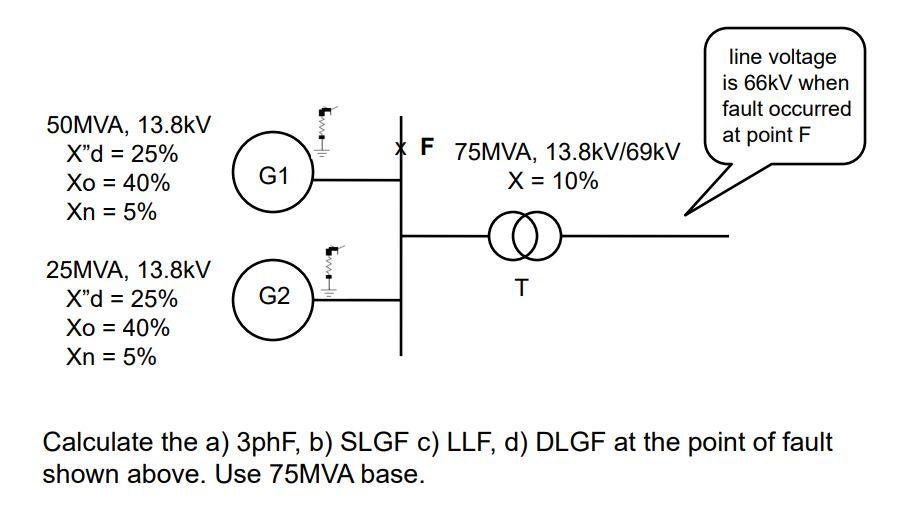# Question Solved1 Answerline voltage is 66kV when fault occurred at point F Uw 50MVA, 13.8kV X”d = 25% Xo = 40% Xn = 5% F 75MVA, 13.8kV/69kV X = 10% G1 = = @ T = G2 25MVA, 13.8kV X"d = 25% Xo = 40% Xn = 5% Calculate the a) 3phF, b) SLGF C) LLF, d) DLGF at the point of fault shown above. Use 75MVA base.COJSPZ The Asker · Electrical EngineeringTranscribed Image Text: line voltage is 66kV when fault occurred at point F Uw 50MVA, 13.8kV X”d = 25% Xo = 40% Xn = 5% F 75MVA, 13.8kV/69kV X = 10% G1 = = @ T = G2 25MVA, 13.8kV X"d = 25% Xo = 40% Xn = 5% Calculate the a) 3phF, b) SLGF C) LLF, d) DLGF at the point of fault shown above. Use 75MVA base.
More
Transcribed Image Text: line voltage is 66kV when fault occurred at point F Uw 50MVA, 13.8kV X”d = 25% Xo = 40% Xn = 5% F 75MVA, 13.8kV/69kV X = 10% G1 = = @ T = G2 25MVA, 13.8kV X"d = 25% Xo = 40% Xn = 5% Calculate the a) 3phF, b) SLGF C) LLF, d) DLGF at the point of fault shown above. Use 75MVA base.